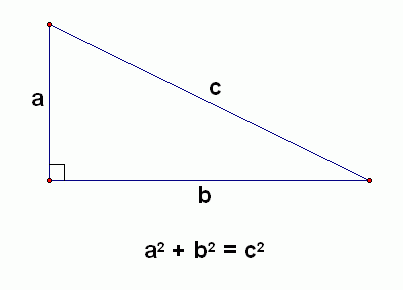﻿ the Pythagorean Theorem
THE PYTHAGOREAN THEOREM
by Dr. Carol JVF Burns (website creator)
Follow along with the highlighted text while you listen!
• PRACTICE (online exercises and printable worksheets)

A 90° angle is called a right angle.
A right triangle is a triangle with a 90° angle.

In a right triangle, the side opposite the 90° angle is called the hypotenuse
and the remaining two sides are called the legs.

The angles in any triangle add up to 180°.

In any triangle, the longest side is opposite the largest angle,
and the shortest side is opposite the smallest angle.

Thus, in a right triangle, the hypotenuse is always the longest side.

The Pythagorean Theorem gives a beautiful relationship between the lengths of the sides in a right triangle:
the sum of the squares of the shorter sides is equal to the square of the hypotenuse.
Furthermore, if a triangle has this kind of relationship between the lengths of its sides, then it must be a right triangle!

 THE PYTHAGOREAN THEOREM Let $\,\,T\,\,$ be a triangle with sides of lengths $a$, $b$, and $c$, where $\,c\,$ is the longest side (if there is a longest side). Then, $T\,$ is a right triangle     if and only if     $a^2 + b^2 = c^2\,$.Have fun with many proofs of the Pythagorean Theorem!
This applet (you'll need Java) is one of my favorites. Let it load, then keep pressing ‘Next’.
EXAMPLES:
Question: Suppose that two angles in a triangle are 60° and 30°.
Is it a right triangle? Answer YES, NO, or MAYBE.
Solution: Yes.
The third angle must be 180° - 60° - 30° = 90° .
Question: Suppose that a triangle has a 100° angle.
Is it a right triangle? Answer YES, NO, or MAYBE.
Solution: No.
The remaining two angles must sum to 80°, so neither remaining angle is a 90° angle.
Question: Suppose that a triangle has a 70° angle.
Is it a right triangle? Answer YES, NO, or MAYBE.
Solution: Maybe.
The remaining two angles must sum to 110°, so one of the remaining angles could be a 90° angle.
Question: Suppose the legs of a right triangle have lengths $\,3\,$ and $\,x\,$,
and the hypotenuse has length $\,5\,$. Find $\,x\,$.
Solution:
$3^2 + x^2 = 5^2$
$9 + x^2 = 25$
$x^2 = 16$
$x = 4$
Note:   $x\,$ cannot equal $\,\text{-}4\,$, because lengths are always positive.
The 3-4-5 TRIANGLE is a well-known right triangle.
Multiplying all the sides of a triangle by the same positive number does not change the angles.
Thus, if you multiply the sides of a 3-4-5 triangle by any positive real number  k ,
then you will still have a right triangle.
For example, these are all right triangles:
 6-8-10 ( k = 2 ) 9-12-15 ( k = 3 ) 1.5-2-2.5 ( k = 0.5 ) 3π-4π-5π ( k = π )
and so on!
Question: Suppose a triangle has sides of lengths $1\,$, $\sqrt{3}$, and $2\,$.   Is it a right triangle?
Solution: YES.
Since $2 > \sqrt{3}\,$, the longest side has length 2.
And, $\cssId{s76}{1^2 + {(\sqrt{3})}^2} \cssId{s77}{= 1 + 3} \cssId{s78}{= 4} \cssId{s79}{= 2^2}\,$.
Master the ideas from this section

When you're done practicing, move on to:
the Distance Formula

CONCEPT QUESTIONS EXERCISE: